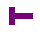# Program BEEST

This program for the Acorn Atom counts the number of possible Hamilton paths from a certain starting point to all other points.

The program consists of two parts. The second part contains the assembly instructions that generate the core algorithm for finding the Hamilton path. After the secons part is ran, it runs the first part, which contains the interface part of the program. This asks for parameters: length, height x-pos (1..length) and y-pos (1..height) of the starting position. While the program is running, the screen is updated, as the counters for the numbers of hamilton paths to each point are stored in the video RAM. After all the solutions are found, the results are printed in matrix form.

For the input: 6 6 1 1, it outputs

```      0   1072      0   1095     0   1770
1072      0   1420      0  1770      0
0   1420      0   1240     0    935
1095      0   1240      0  1180      0
0   1770      0   1180     0    590
1770      0    935      0   590      0

TOTAAL :   22144
```

## Part 1

Saved as KL BEEST P1 from 8200-8300.
```  190G.d
201dIN."L"L,H,A,B
220F.I=0TOL;F.J=1TOH;F?(I+Z*J)=32;N.;N.
230F.I=0TO L+1;F?I=V;F?(I+H*Z+Z)=V;N.
240F.I=0TO H+1;F?(I*Z)=V;F?(1+L+I*Z)=V;N.
250 ?K=L*H-1;I=#83;?I=0;?C=A+B*Z;F?(?C)=V
254P.\$12
270A=9472;F.I=#8000TO#81FF;I?A=?I;N.
280F.I=0TOL;F.J=1TOH
271P.\$12;@=7;T=0
290B=#A500+I+Z*J;B=?B+(B?#100)*256-8224;P.B;T=T+B;N.;P.';N.
295P.''"TOTAAL :"T
270aIF?I=#FF G.b
```

## Part 2

Saved as KL BEEST P2 from A000-A200.
```   10C=#80;D=#81;E=#82;I=#83
20K=#84;R=#85;V=42;L=32
30F=#A500;S=#A600
31REMZ=16;!R=#FFF00110
32   Z=32;!R=#FFE00120
50tDIMLL9;F.J=0TO1;P=#A700
60[LDX@0;LDA@L
65:LL0 STA#8000,X;STA#8100,X;INX;BNE LL0;LDA C
70:LL1
73 TAX;LDA F+1,X;CMP F-1,X;BNE LL7
74 LDA F+Z,X;CMP F-Z,X;BNE LL7
75 TXA; JMP LL4
79:LL7 TXA;LDY@0
90LDA@V;STA F,X;STX C;LDX I;TYA;STA S,X; INC I;LDA C
100LDX I;CPX K;BNE LL1
105 TAX;INC#8000,X;BNE LL4;INC #8100,X
110:LL4 DEC I;LDX I;CPX@#FF; BNE LL5;RTS
120:LL5 LDY S,X;TAX;LDA@L;STA F,X
130:LL6 TXA;SEC;SBC R,Y;INY;CPY @4;BNE LL2;BEQ LL4
150];N.;Q=LL3
200 ?18=#82;G.d
```

## Input file for Acorn Atom Emulator

If you want to run this program on the Acorn Atom Emulator, you can try loading the saved source of this HTML file.
hacker page | counting page | Acorn Atom Emulator page